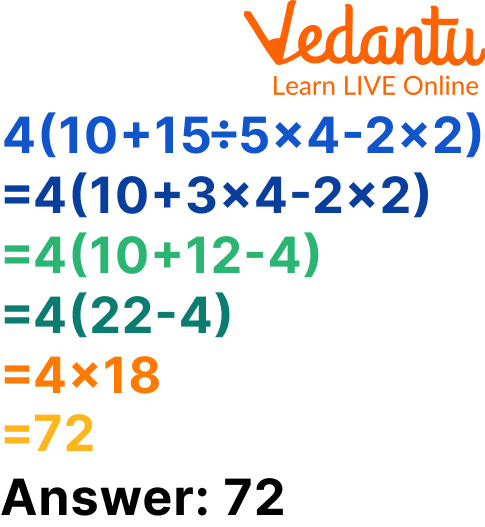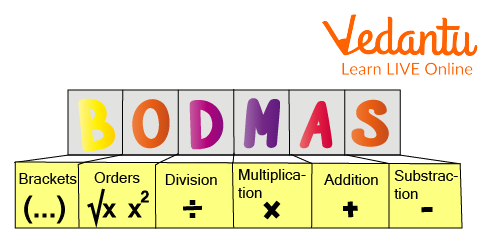Courses
Courses for Kids
Free study material
Free LIVE classes
More

# Simplify QuestionsLIVE
Join Vedantu’s FREE Mastercalss

## Let’s Learn Some Interesting Simplify Questions

Do you feel lost when it comes to Maths problems? If that's the case, simplification questions happen to be your best friend. Join us as we walk you through this problem-solving technique, giving an example of how they work and how they can help simplify Maths examples that are difficult concepts to solve!

Simplification questions can be useful because they help you to solve more complicated problems with ease. Simplification questions are asked in Maths to help the learner simplify a concept. They can be used to help in getting rid of complications. You are simplifying so they can understand the reasoning behind the question.

## What are Simplification Questions?

The word simplification refers to any process of making something as easy as possible. Simplification questions are questions that can simplify Maths examples, like the one below:

Example: Simplify $4(10+15\div\,5\times 4-2\times 2)$

Ans: The answer is: $72$Simplify the Question

The basic idea behind simplifying is that you want to transform your number or expression into its simplest form by reducing the numerical value and getting rid of the signs.

## What is the Simplification Formula?

BODMAS: This is an acronym for a set of steps that you should follow when answering a question, which are: Bracket, Order, Division, Multiplication, Addition, and Subtraction. The objective is to reduce the number as much as possible without causing problems with addition or subtraction.

• B

• O: Operation

• D: Division

• M: Multiplication

• S: SubtractionBODMAS

## How Do You Solve Simplification Questions?

To solve simplification problems, you must understand the process behind simplifying.

• Step 1: Check for the operations that are equal to each other.

Example: Simplify (a+bc)+(b-d)(a-c)(d+b)-2, using the BODMAS simplification formula.

• Step 2: Check for similar operations of the operands. Example: Simplify (ab+cd)(ab-bc)(a-b)+(c-d).

• Step 3: If operations are not equal or similar, simplify the one on the right of the equal sign first.

• Step 4: Add together (or multiply) all terms using the same or similar operations. Example: Simplify x(x+3)+(x-9)

• Step 5: Include any brackets with numbers that have been added together (or multiplied).

## How Do You Simplify a Fraction?

You can combine the numerator and the denominator by adding, subtracting, or multiplying them together.

• For example, one of the simplification questions with solutions is, $\dfrac{12}{6}$ simplifies to $\dfrac{2}{3}$ by adding 6+12 to get 18 and $\dfrac{18}{3}$=6.

• For example, 5x4=20 becomes $\dfrac{20}{10}$= 2 so 5x4 = 20 or 2x5 = 10.

The new number is now divided into 10 parts instead of four parts to get your final answer of two.

## How to Simplify Decimals?

If a number is in decimals, you would have to convert it into fractions before simplifying. The simplest form of converting $\dfrac{2}{3}$ into a decimal which is 0.66 or to a fraction with one unit, $\dfrac{6}{1}$=6 becomes the answer.

If it were in fractions, then your number would be easier than doing the multiplication. So try converting them into fractions first, and then simplify while solving simplification problems.

## Solved Examples

Q1 Simplify: 37 - [5 + {28 - (19 - 7)}]

Ans: 37 - [5 + {28 - (19 - 7)}]

= 37 - [5 + {28 - 12}] (Removing the innermost bracket ( ))

= 37 - [5 + 16]

= 37 - 21

= 16.

Q2 Simplify: 78 - [24 - {16 (5 - 4 - 1)}]

Ans:-78 - [24 - {16 (5 – 4 - 1)}]

= 78 - [24 - {16(5 - 5)}] (Removing vinculum)

= 78 -[24 - {16 (0)}] (Removing parentheses)

= 78 - [24 – 0] (Removing braces)

= 78 - 24

= 54

Q3. Simplify. $\dfrac{1}{3}+\left[\dfrac{1}{2}-\left\{\dfrac{1}{5}+\left(\dfrac{1}{3}-\dfrac{1}{5}\right)\right\}\right]$

Ans: $\dfrac{1}{3}+\left[\dfrac{1}{2}-\left\{\dfrac{1}{5}+\left(\dfrac{1}{3}-\dfrac{1}{5}\right)\right\}\right]$

$=\dfrac{1}{3}+\dfrac{1}{6}$

$= \dfrac{2+1}{6}$

$=\dfrac{1}{2}$

## Practice Questions

Q1. 3 - (5 – 6 ÷ 3)

Ans: 0

Q2. – 25 + 14 ÷ (5 - 3)

Ans: -18

Q3. 25-{5+4-(3+2-1+3)}

Ans: 23

Q4. 27 - [38 - {46 - (15 - 13 - 2)}]

Ans: 35

Q5 36 - [18 - {14 - (15 – 4 ÷ 2 x 2)}]

Ans: 21

## Summary

We've covered a lot of different strategies for simplification questions in this article. We've focused on the BODMAS method, which is a helpful way to simplify original equations, and learned to simplify equations by cancelling out factors.

In our opinion, it is one of the ways to learn maths quickly, because you can use this rule over and over again. At first, you do not understand what it truly means, but as you keep applying it in real-life situations and play games, you begin to grasp the meaning of how many even numbers there are in a set of numbers.

Last updated date: 23rd Sep 2023
Total views: 114.3k
Views today: 2.14k

## FAQs on Simplify Questions

1. How many types of Simplification Questions are there?

There are four fundamental types, split into four different core concepts. The first one is decomposing a problem into parts. The second is calculating with addition and subtraction; this includes dividing multi-digit numbers and fractions into small pieces by elimination or division. The third is simplifying linear equations by rearranging the original equation or using the BODMAS framework. Lastly, we will discuss simplifying square roots using the BODMAS formula, which we learned above: Brackets, Operation, Division, Multiplication, Addition, and Subtraction, in the same order.

2. What is the meaning of simplifying in Maths?

The meaning of simplification in Maths is to reduce an equation or fraction in order to make it simple.

3. Why are simplification questions practical?

Anything simplified refers to a process that makes anything as simple as feasible. Math questions that can be simplified are known as simplification questions, thus making Math easy for all. Simplification is considered to be the most important part of bank exams. Though the number of questions from this part is limited, its usefulness in all quantitative parts is immense. Simplification helps develop much-needed speed in the real exam. The questions from this section are generally simple, where you have to fill in a missing expression or a missing value.